# Galvanometer

Definition: The galvanometer is the device used for detecting the presence of small current and voltage or for measuring their magnitude. The galvanometer is mainly used in the bridges and potentiometer where they indicate the null deflection or zero current.

Principle: The potentiometer is based on the premise that the current sustaining coil is kept between the magnetic field experiences a torque.

## Construction of the Galvanometer

The construction of the potentiometer is shown in the figure below.The moving coil, suspension, and permanent magnet are the main parts of the galvanometer.

Moving Coil – The moving coil is the current carrying part of the galvanometer. It is rectangular or circular and has the number of turns of fine copper wire. The coil is freely moved about its vertical axis of symmetry between the poles of a permanent magnet. The iron core provides the low reluctance flux path and hence provides the strong magnetic field for the coil to move in.

Suspension – The coil is suspended by a flat ribbon which carries the current to the coil. The other current carrying coil is the lower suspension whose torque effect is negligible. The upper suspension coil is made up of gold or copper wire which is made in the form of a ribbon. The mechanical strength of the wire is not very strong, and hence the galvanometers handle carefully without any jerks.

Mirror – The suspension carries a small mirror which casts the beam of light. The beam of light placed on the scale on which the deflection is measured.

Torsion Head – The torsion head is used for controlling the position of the coil and for adjusting the zero setting.

## Applications of Galvanometer

The galvanometer has following applications. They are

• It is used for detecting the direction of current flows in the circuit. It also determines the null point of the circuit. The null point means the situation in which no current flows through the circuit.
• It is used for measuring the current.
• The voltage between any two points of the circuit is also determined through galvanometer.

### Working of Galvanometer

Let, l, d – the length of respective vertical and horizontal side of the coil in the meter.
N – number of turns in the coil,
B – Flux density in the air gap, wb/m2
i – current through moving coil in Ampere
K – spring constant of suspension, Nm/rad
When the current flows through the coil, it experiences a torque which is expressed asThe force on each side of the coil is given as,Where,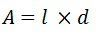N, B, A  are the constant of the galvanometer.The G is called the displacement constant of the galvanometer, and their value is equal NBA = NBld.

The controlling torque exerted by the suspension at deflection θF is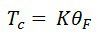For final steady deflection,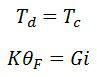Hence final steady deflection,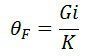For the small deflection angle, the deflection is expressed as the product of the radius and angle of the turned. By the reflected beam, it is expressed as 1000 Χ 2θF = 2000 Gi / K in millimetre.

The above equation shows that when the mirror turns through an angle θF the reflected beam turns through an angle 2θF shown in the figure below.

###Conversion of Galvanometer into an Ammeter

The galvanometer is used as an ammeter by connecting the low resistance wire in parallel with the galvanometer. The potential difference between the voltage and the shunt resistance are equal.

###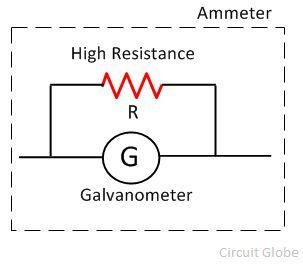Where, S = shunt resistance and Is = current across the shunt.

As the galvanometer and the shunt resistance are connected in potential with the circuit, their potentials are equal.Thus, the shunt resistance is given as,The value of the shunt current is very small as compared to the supply current.

### Conversion of Galvanometer into a voltmeter

The galvanometer is used as a voltmeter by connecting the high resistance in series with the circuit.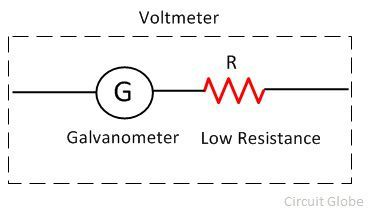###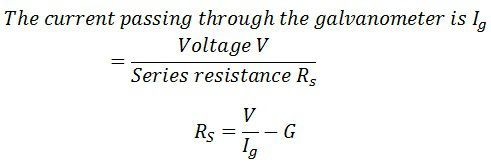The range of the voltmeter depends on the value of the resistance connected in series with the circuit.

### 5 thoughts on “Galvanometer”

1. why in the diagram low series resistance in case of voltmeter and high resistance incase of ammeter